Article Outline

# 函数

• 它的输入是怎么构成的（都有哪些参数？如何指定？）；
• 以及它的输出是什么（返回值究竟是什么？）……

NOTE

https://docs.python.org/3/library/functions.html

## 示例 print()

### 基本的使用方法

`print()` 是初学者最常遇到的函数 —— 姑且不说是不是最常用到的。

``````print('line 1st')
print('line 2nd')
print()
print('line 4th')``````
``````line 1st
line 2nd

line 4th``````

``print('Hello,', 'jack', 'mike', '...', 'and all you guys!')``
``Hello, jack mike ... and all you guys!``

``````name = 'Ann'
age = '22'
print(f'{name} is {age} years old.')``````
``Ann is 22 years old.``

``````name = 'Ann'
age = '22'
a = f'{name} is {age} years old.'
print(a)``````
``'Ann is 22 years old.'``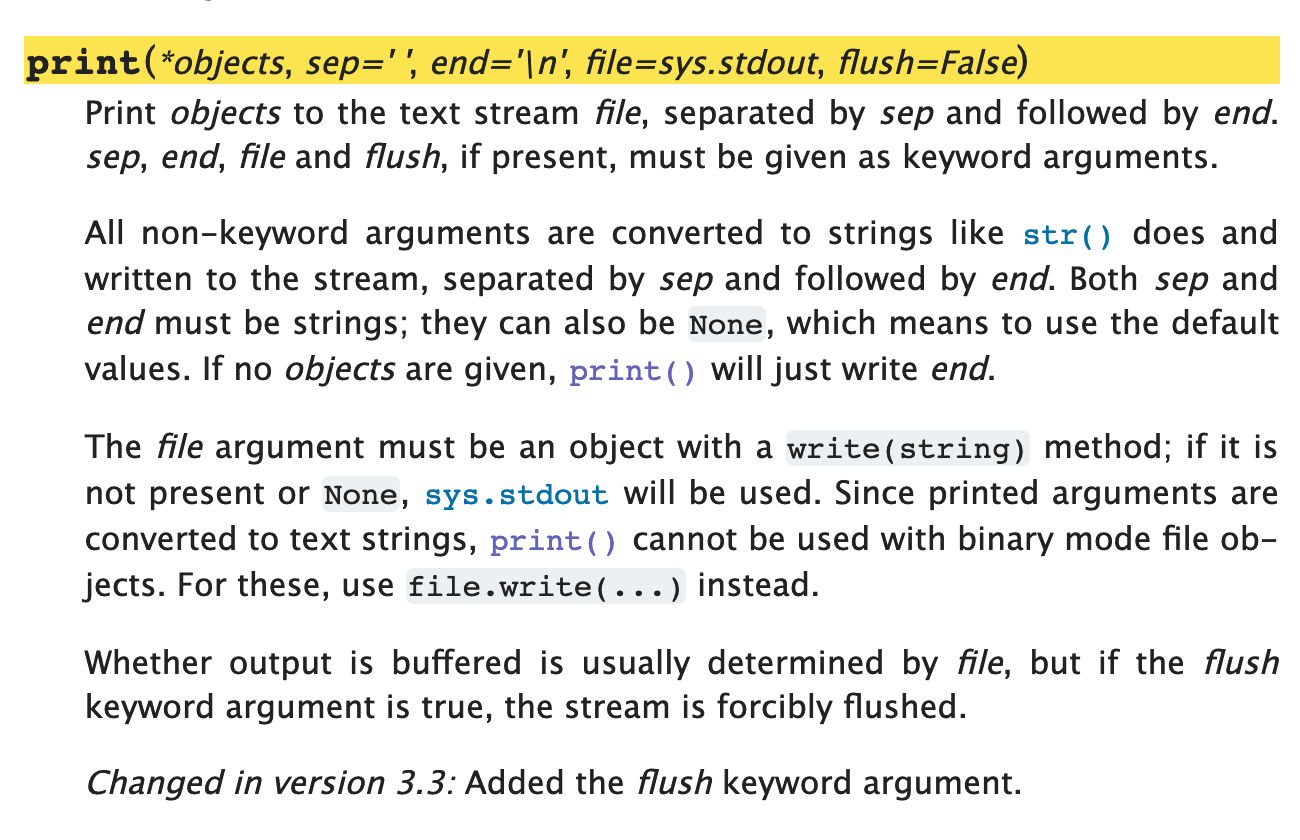`print(*object, sep=' ', end='\n', file=sys.stdout, flush=False)` 20

• `sep=' '`：接收多个参数之后，输出时，分隔符号默认为空格，`' '`
• `end='\n'`：输出行的末尾默认是换行符号 `'\n'`
• `file=sys.stdout`：默认的输出对象是 `sys.stdout`（即，用户正在使用的屏幕）……

``````import sys                                  # 如果没有这一行，代码会报错

print('Hello', 'world!')                    # 下一行的输出和这一行相同
print('Hello', 'world!', sep=' ', end='\n', file=sys.stdout, flush=False)
print('Hello', 'world!', sep='-', end='\t')
print('Hello', 'world!', sep='~')           # 上一行的末尾是 \t，所以，这一行并没有换行显示
print('Hello', 'world!', sep='\n')          # 参数之间用换行 \n 分隔``````
``````Hello world!
Hello world!
Hello-world!    Hello~world!
Hello
world!``````

`print()` 这个函数的返回值是 `None` —— 注意，它向屏幕输出的内容，与 `print()` 这个函数的返回值不是一回事。

``print(print(1))``
``````1
None``````

## 关键字参数

• 位置参数（Positional Arguments，在官方文档里常被缩写为 arg
• 关键字参数（Keyword Arguments，在官方文档里常被缩写为 kwarg

`sorted(iterable, *, key=None, reverse=False)`

``````a = sorted('abdc')
b = sorted('abdc', reverse=True)
print(a)
print(b)``````
``````['a', 'b', 'c', 'd']
['d', 'c', 'b', 'a']``````

## 位置参数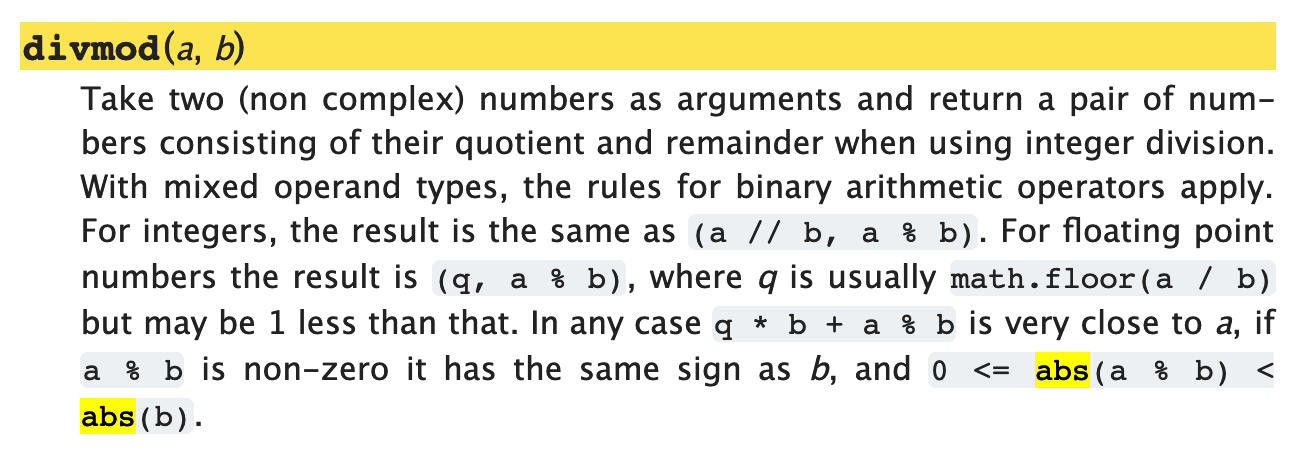• 当你调用这个函数的时候，括号里写的第一个参数，是被除数，第二个参数是除数 —— 此为该函数的输入；
• 而它的返回值，是一个元组（Tuple，至于这是什么东西，后面讲清楚），其中包括两个值，第一个是商，第二个是余 —— 此为该函数的输出。

``````print(divmod(11, 3))
a, b = divmod(11, 3)
print(a)
print(b)

print(divmod(3, 11))
a, b = divmod(3, 11)
print(a)
print(b)``````
``````(3, 2)
3
2
(0, 3)
0
3``````

## 可选位置参数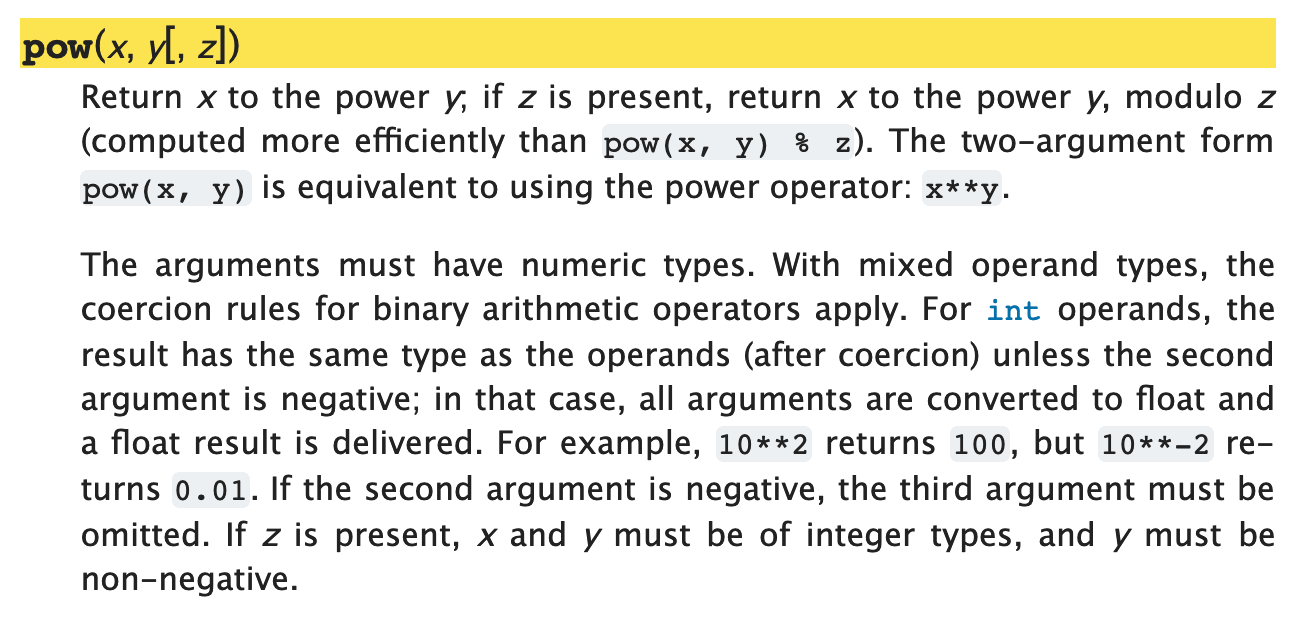• `pow(x, y)` —— 返回值是 `x ** y`
• `pow(x, y, z)` —— 返回值是 `x ** y % z`
``````print(pow(2, 3))
print(pow(2, 3, 4))``````
``````8
0``````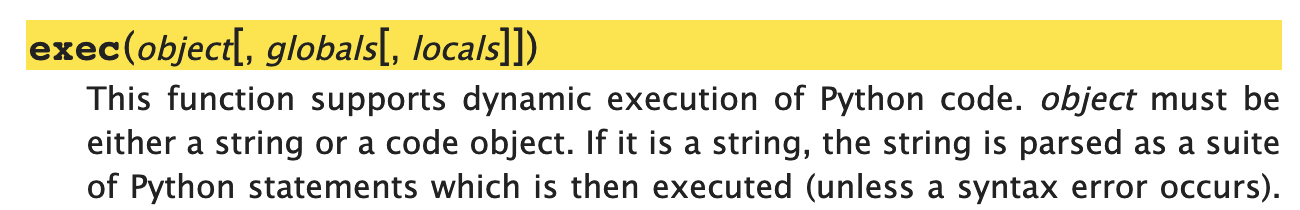• 没在方括号里的 `object` 是不可或缺的参数，调用时必须提供；
• 可以有第二个参数，第二个参数会被接收为 `globals`
• 在有第二个参数的情况下，第三个参数会被接收为 `locals`
• 但是，你没办法在不指定 `globals` 这个位置参数的情况下指定 `locals`……

## 可接收很多值的位置参数## Class 也是函数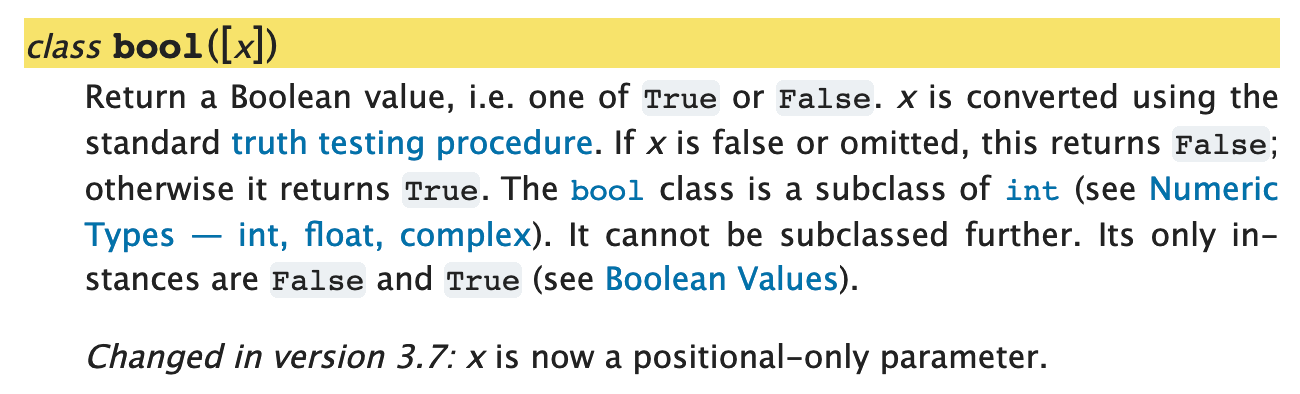``````print(bool())
print(bool(3.1415926))
print(bool(-3.1415926))
print(bool(1 == 2))
print(bool(None))``````
``````False
True
True
False
False``````

## 总结

• 你可以把函数当作一个产品，而你自己是这个产品的用户；
• 既然你是产品的用户，你要养成好习惯，一定要亲自阅读产品说明书；
• 调用函数的时候，注意可选位置参数的使用方法关键字参数的默认值
• 函数定义部分，注意两个符号就行了，`[]``=`
• 所有的函数都有返回值，即便它内部不指定返回值，也有一个默认返回值：`None`
• 另外，一定要耐心阅读该函数在使用的时候需要注意什么 —— 产品说明书的主要作用就在这里……

1. ^ `print()` 函数的官方文档里，`sep=''` 肯定是 `sep=' '` 的笔误 —— 可以用以下代码验证（写于 2019.02.14）：
``````print('a', 'b', sep='')
print('a', 'b')``````

2019.03.16, 有读者提醒：https://github.com/selfteaching/the-craft-of-selfteaching/issues/111